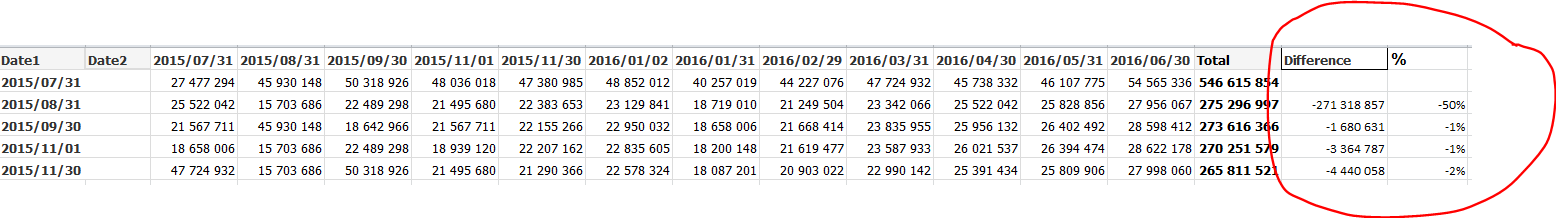# New to QlikView

Discussion board where members can get started with QlikView.

HighlightedNot applicable

## Calculation on Partial Sum

Greetings

Please help to calculate the difference of the partial sum on Pivot table.Please advise how can I calculate the difference between the sum of 1st column vs 2nd column and so on on a Pivot Table.

Thanks!!

Tags (2)
1 Solution

Accepted Solutions
Valued Contributor III

## Re: Calculation on Partial Sum

HI,

t

use above function

Ex:

Sum(sales)-above(sum(sales))

7 Replies
Valued Contributor III

## Re: Calculation on Partial Sum

HI,

t

use above function

Ex:

Sum(sales)-above(sum(sales))Not applicable

## Re: Calculation on Partial Sum

Thanks Sunny!

But unfortunately it's not working.

Please find attach the qvw file.

Thanks.

Valued Contributor III

## Re: Calculation on Partial Sum

Hi,

PFA , see the last columnNot applicable

## Re: Calculation on Partial Sum

Thanks Sunny.

Is it possible if only last column will be displayed?

Thanks.

Valued Contributor III

## Re: Calculation on Partial Sum

HI,

i don`t think so , it will apply on all columnsMVP

## Re: Calculation on Partial Sum

By last column you mean just the totals column?Not applicable

## Re: Calculation on Partial Sum

Yes. Just the total column.

Thanks.